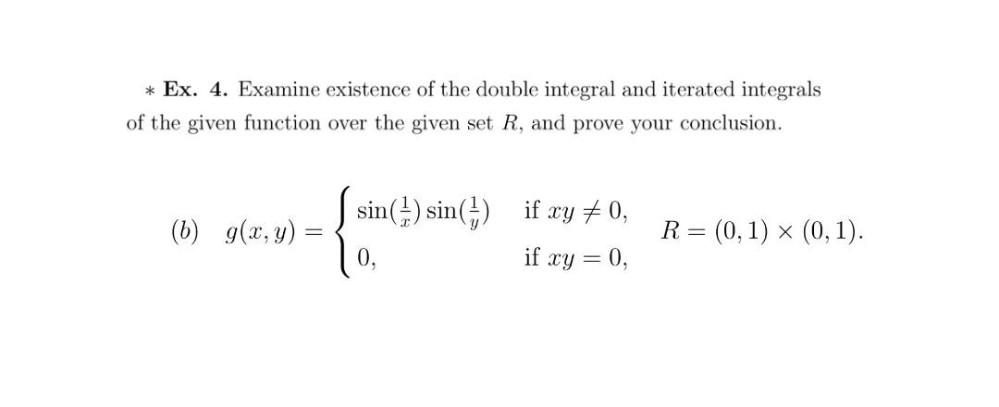Question:

# * Ex. 4. Examine existence of the double integral and iterated integrals of the given function over the given set R, and prove y* Ex. 4. Examine existence of the double integral and iterated integrals of the given function over the given set R, and prove your conclusion. (6) g(x,y) - Leshrane sin(1) sin(1) if xy +0, if xy = 0, R= = (0,1) > (0, 1). x =